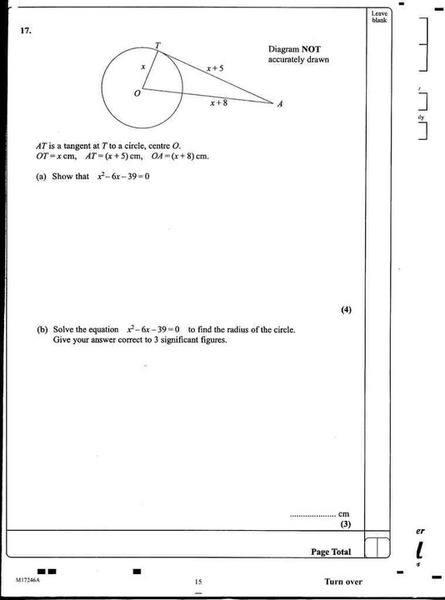# Calculator, Q 17 - what it getting atWhat is this question going for. I can identify that OTA is a right angled triangle..... Where do I go from

cristo
Staff Emeritus
What is this question going for. I can identify that OTA is a right angled triangle..... Where do I go from
Pythagoras?

$$(x+8)(x+8) = x^{2} + (x+5)(x+5)$$

$$x ^ {2} + 16x + 64 = x^4+10x+25$$

take LHS from RHS

$$x^{2} - 6x - 39 = 0$$

but how did you know to use pythagerous?

arildno
Homework Helper
Gold Member
Dearly Missed
What relationships DO you know hold for right-angled triangles that might come in handy?

cristo
Staff Emeritus
$$(x+8)(x+8) = x^{2} + (x+5)(x+5)$$

$$x ^ {2} + 16x + 64 = x^4+10x+25$$

take LHS from RHS

$$x^{2} - 6x - 39 = 0$$
Sorry, one correction to your second line.

$$x ^ {2} + 16x + 64 = 2x^2+10x+25$$

but how did you know to use pythagerous?

Well, you did the hard part; spotting that it was a right angled triangle. Pythagoras' theorem holds for right angled triangles, and is a relationship relating the squares of the sides. Since the solution contains an x^2, this is quite a big hint as to what you should use.